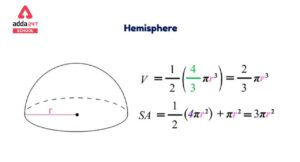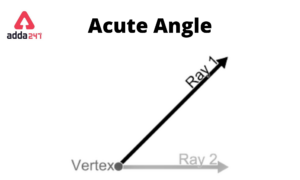Online Tution   »   Important Question   »   Perimeter of Triangle- Formula, Definition, and...

# Perimeter of Triangle- Formula, Definition, and Examples

## Perimeter of Triangle

Perimeter of triangle is P= a+b+c, where a,b,c are the sides of triangles. Any two-dimensional figure’s perimeter is defined as the distance around it. By combining the lengths of each of the sides, we can compute the perimeter of any closed shape. In this tutorial, you will learn what the perimeter is and how to calculate the perimeter of various types of triangles when all side lengths are known. Furthermore, the solved examples will assist you in gaining additional perspectives on the subject.

## Perimeter of Triangle- Definition

Any polygon’s perimeter is equal to the sum of its side lengths.

 Perimeter = Sum of the three sides in the case of a triangle

In your final answer, always mention units. If the triangle’s sides are measured in cm, the final result should be in centimeters as well.

## Perimeter of Triangle- Formula

In most cases, the perimeter of a closed shape figure is equal to the length of the figure’s outer line. As a result, the perimeter of a triangle is equal to the total of its three sides. If a triangle has three sides, a, b, and c, then,

 Perimeter, P = a + b +c

## Perimeter of Different Kinds of Triangle

The perimeter of a triangle can be calculated by adding the lengths of the three sides of triangle. But there are different methods as well to calculate to perimeter of different types of triangles.

## Perimeter of Isosceles Triangle

Because two sides of an Isosceles triangle are equal and one side is of different length than the other two, therefore the perimeter of an isosceles triangle can also be calculated by the formula:

 Perimeter, P= (2 × A) + B

Where A is the length of two equal sides and B is the length of the third side.

## Perimeter of Equilateral Triangle

Because all the sides of a equilateral triangle are equal to each other, therefore the perimeter of a equilateral triangle can also be calculated by the formula:

 Perimeter, P= 3 × L

Where L is the length of the sides of triangle.

## Perimeter of Scalene Triangle

Because the sides of a scalene triangle are all of different lengths, therefore it can only be calculated by the conventional formula:

 Perimeter, P= A + B + C

Where A, B, C are the lengths of the sides of a triangle.

## Perimeter of Triangle- Examples

Example 1: Find the perimeter of a triangle whose sides are 7 cm, 8 cm and 5 cm.

Solution: Because, all three sides are unequal, therefore it is a scalene triangle, and we can only calculate the perimeter by the conventional method. I.e. P=A+B+C.

A = 7 cm

B = 8 cm

C= 5 cm

Perimeter = Sum of all sides = A + B + C = 7 + 8 + 5 = 20

Therefore, the answer is 20 cm.

Example 2: Find the perimeter of a triangle which has each side is 15 cm.

Solution: Because all the three sides are equal in length, the triangle given is an equilateral triangle, and hence the perimeter can be calculated by the formula: P = 3 × L.

Here, L = 15 cm

Therefore, Perimeeter = 3 × 15 = 45

Therefore, Perimeter of the given triangle is 45 cm.

Example 3: What is the length of the third side of a triangle whose perimeter is 50 cm and two sides are 15 cm and 12 cm?

Solution: Given,

Perimeter, P = 50 cm

Using formula: P = A + B + C

A = 15 cm

B = 12 cm

40 = 15 + 12 + C

C = 50 – 27 = 23

Missing side length is 23 cm.

Example 4: Calculate the Perimeter of a Right Triangle with Base as 6 cm and height as 8 cm.

First, using the Pythagorean Theorem, we can calculate the hypotenuse of the right triangle.

h =√(base2+ height2)

h = √(62+82)

h = √(36 + 64)

h = √100

Or, h = 10 cm

So, the perimeter of the triangle = 6 + 8 + 10 = 24 cm.

Related Post:

## Triangle Perimeter Formula- FAQs

What does the Perimeter of a Triangle Mean?

The total distance around a triangle’s edges is known as its perimeter. In other terms, the perimeter of a triangle is the length of its boundary.

How to Calculate the Perimeter of a Triangle?

Add the lengths of the triangle’s sides to find its perimeter. For instance, if a triangle has sides a, b, and c, then its perimeter is P = a + b + c.

What is the formula of area and perimeter of Triangle?

The formula for calculating the area of a triangle is: A = 1/2 (b × h). The perimeter of a triangle can be calculated by adding the lengths of all the three sides of the triangle. P = A + B + C.

Sharing is caring!

## FAQs

### What does the Perimeter of a Triangle Mean?

The total distance around a triangle's edges is known as its perimeter. In other terms, the perimeter of a triangle is the length of its boundary.

### How to Calculate the Perimeter of a Triangle?

Add the lengths of the triangle's sides to find its perimeter. For instance, if a triangle has sides a, b, and c, then its perimeter is P = a + b + c.

### What is the formula of area and perimeter of Triangle?

The formula for calculating the area of a triangle is: A = 1/2 (b × h). The perimeter of a triangle can be calculated by adding the lengths of all the three sides of the triangle. P = A + B + C.

### TOPICS:

•Surface Area of Sphere- Formula, Derivat...
•Scattering of light Examples, Definition...
•GDP: सकल घरेलू उत्...
•National Tree of India Name is Banyan Tr...
•Acute angle: Definition, Degree, Triangl...
•Scientific Name of Goat in India- Pronun...